# A guide to LSTMs(Long Short Term Memory Networks)Photo by Inja Pavlić on Unsplash

This is my first article on any thing to do with Neural Networks and I’m super excited to share what I have learnt so far. Lets dive in

### Recurrent Neural Networks(RNNs)

Lets say we have a sentence “The cat, which already ate was full” it is clear that “was” in the sentence is dependent on whether “cat” is singular or plural. If it was made plural the sentence would be like “The cats, which already ate were full”. In order to build a model for this case we need information from previous words in the sentence.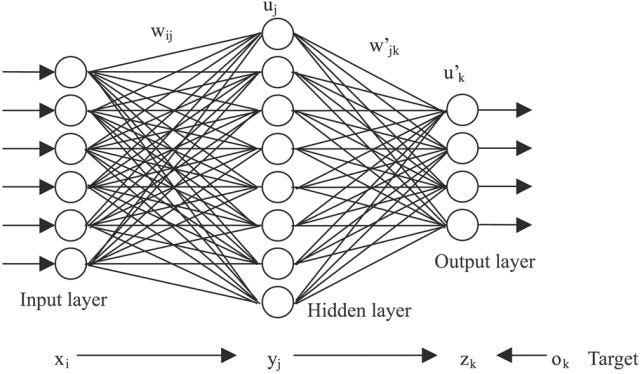Neural network with one hidden layer

Traditional neural networks can’t do this as they don’t use information from previous events to predict the future events. Recurrent neural networks seek to solve this issue.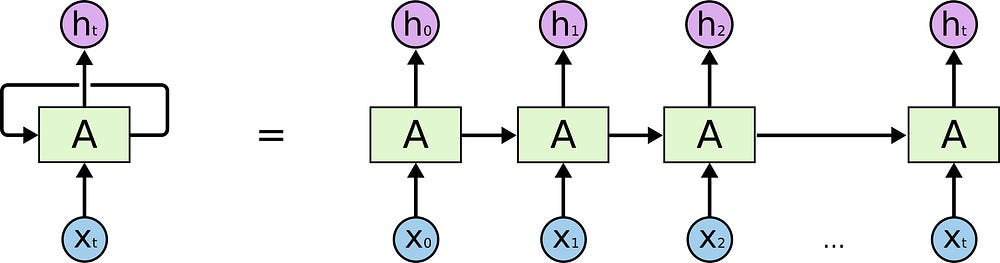Recurrent Neural Network

The unrolled recurrent neural network here shows a dependence of the next output in the sequence on the previous inputs in the sequence. This allows us build language models where we predict the next word based on previous ones.

Recurrent neural networks seem to solve the problem of dependence on previous information, but there lies a fundamental problem when the network has long range dependencies, for example longer sentences or even a paragraph.

Recurrent neural networks suffer from vanishing gradients and exploding gradients. The latter problem is easily solved with gradient clipping which is caused by overshooting gradients. Gradient clipping solves this issue by setting a threshold to prevent the gradient from overshooting.

However the vanishing gradient problem as we will see shortly is solved by certain variations of RNNs. The vanishing gradient problem is problem found with most gradient based learning approaches especially very deep networks,the unrolled RNN is very deep. It occurs when the gradients in the earlier layers gets smaller and smaller and tend to “vanish”, a more detailed explanation can be found here. This can be thought as forgetting its past dependencies needed to predict the next word.

### LSTM Networks

The Long Term Short Term Network derives it’s name from a paper written by Sepp Hochreiter et al where Long term refers to the learned weights of the network and Short term refers to cell state. Don’t worry about the details we’ll understand shortly.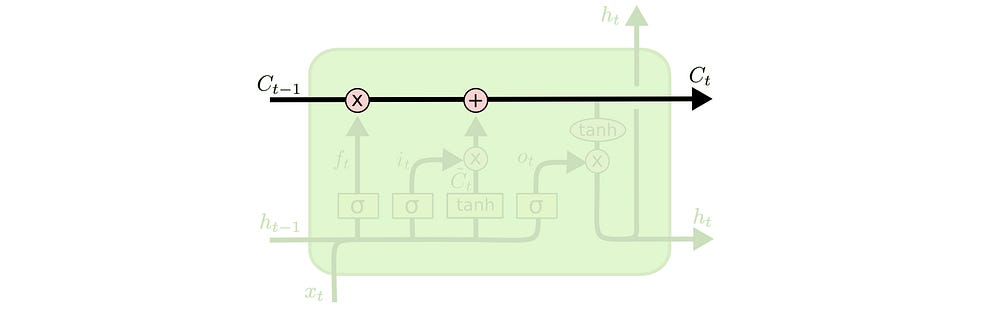Cell state credit: http://colah.github.io/posts/2015-08-Understanding-LSTMs/

The unique characteristic of the LSTM is the ability to control and maintain the cell state. The cell state can be thought of as a copy of the past dependencies, in our sentence example earlier “cat” would be a dependency. It is represented by the long straight black line in the diagram above. Note that the dependencies or state are stored as vectors. So it’s just a vector moving from one unit to another. The cell state are a controlled by three gates.These gates are a sigmoid function applied to a neural network layer. Let’s take a look at them

#### The Forget Gate ft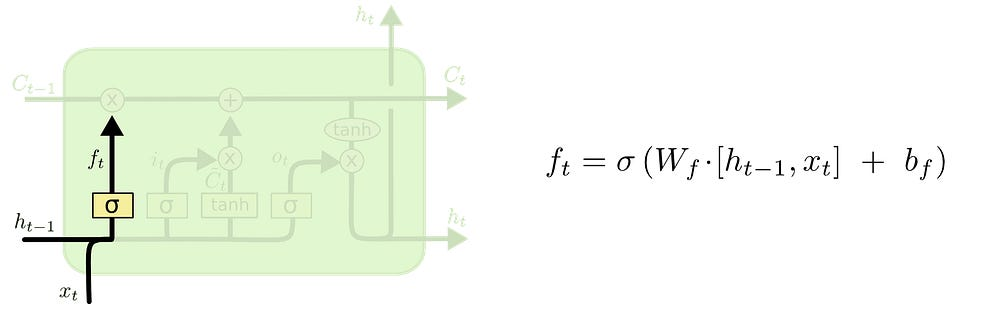Forget gate

This gate as the name implies clears the cell state from the previous LSTM unit. The forget gate equation being a sigmoid function applied to a neural net layer squashes the output of that layer between 0 and 1. This means that if we do a point-wise multiplication of the gate value ft with the previous cell state Ct-1 then we can determine how much we want to remember from the previous cell state. So a gate output of zero would mean remember nothing from the previous units and a gate output of 1 means keep the cell state.

#### The Update Gate

The update gate i_t is used to update the cell state. The update gate is also a sigmoid function applied to neural net layer so values range from 0 to 1. When the lstm unit computes a new value

which is the tanh activation of a layer which we want to update our cell state with. Whether this vector is passed into the cell or not is determined by the update gate. This gives the unit control over the cell state. So a pointwise addition of the new value and an i_t value of 0 means the cell state is not updated with the new value and 1 would mean the cell state is updated with the new value. So there we have it our new cell state which then passed to another lstm unit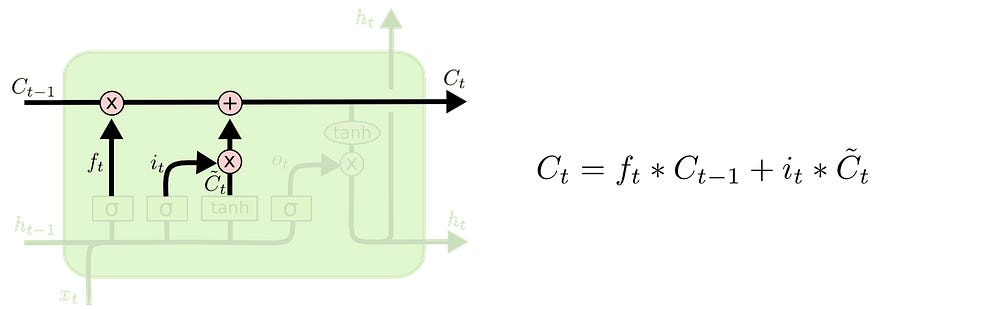Final point-wise addition

#### The Output Gate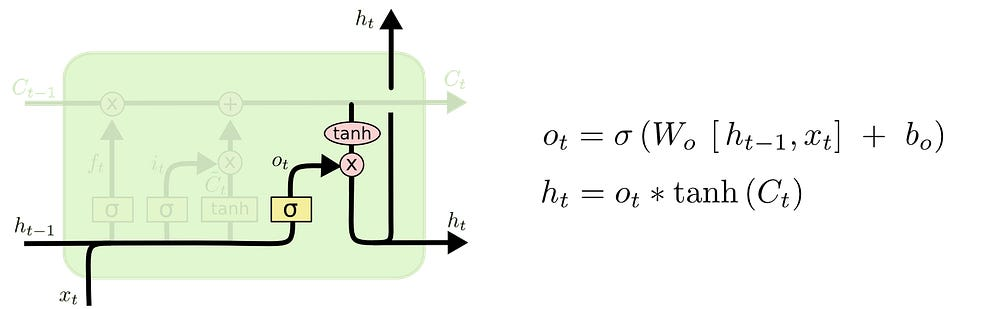Output gate

To determine the output ht we finally apply one sigmoid layer called the output gate. For the output value we apply a tanh to the current cell state to push the values of the output between -1 and 1 and multiply it with the output of the output gate value to get our output value ht.

This article was inspired by this blog post. My next post will be implementing LSTMs from scratch in python. Will be posting soon.

Please leave questions in the comment section. Thank you

Source: Deep Learning on Medium